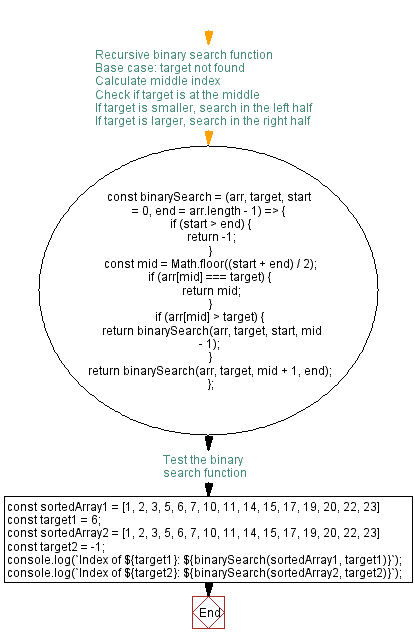# JavaScript: Binary Search Algorithm using recursion

## JavaScript Function: Exercise-12 with Solution

Write a JavaScript program to search for a given integer in an array of sorted integers using the Binary Search Algorithm and recursion.

Test Data:
([1, 2, 3, 5, 6, 7, 10, 11, 14, 15, 17, 19, 20, 22, 23], 6) -> 4
([1, 2, 3, 5, 6, 7, 10, 11, 14, 15, 17, 19, 20, 22, 23], 16) -> -1

Sample Solution:

HTML Code:

``````<!DOCTYPE html>
<html>
<meta charset="utf-8">
<title>Binary Search Algorithm using recursion</title>
<body>

</body>
</html>
```
```

JavaScript Code:

``````/**
* Source: bit.ly/3h77zX6
* @function BinarySearch
* @description Search the integer inside the sorted integers array using Binary Search Algorithm.
* @param {Integer[]} arr - sorted array of integers
* @param {Integer} low - The input integer
* @param {Integer} high - The input integer
* @param {Integer} searchValue - The input integer
* @return {Integer} - return index of searchValue if found else return -1.
*/
const binary_Search = (arr, searchValue, low = 0, high = arr.length - 1) => {
// base case
if (high < low || arr.length === 0) return -1
const mid = low + Math.floor((high - low) / 2)
// If the element is present at the middle
if (arr[mid] === searchValue) {
return mid
}
// If element is smaller than mid, then
// it can only be present in left subarray
if (arr[mid] > searchValue) {
return binary_Search(arr, searchValue, low, mid - 1)
}
// Else the element can only be present in right subarray
return binary_Search(arr, searchValue, mid + 1, high)
}
const myArray = [1, 2, 3, 5, 6, 7, 10, 11, 14, 15, 17, 19, 20, 22, 23];
console.log(binary_Search(myArray, 6));
console.log(binary_Search(myArray, 16));
```
```

Output:

```4
-1
```

Flowchart:Live Demo:

See the Pen javascript-recursion-function-exercise-12 by w3resource (@w3resource) on CodePen.

Improve this sample solution and post your code through Disqus

What is the difficulty level of this exercise?

Test your Programming skills with w3resource's quiz.

﻿